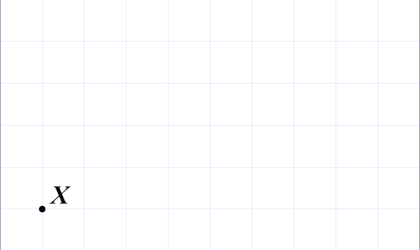# Exam-Style Questions.

## Problems adapted from questions set for previous Mathematics exams.

### 1.

GCSE Higher

Multiply out and simplify:

$$(x-6)^2$$

### 2.

GCSE Higher

Find the highest common factor of the following two expressions:

$$8x^5y^3$$ $$6x^2y$$

### 3.

GCSE Higher

Simplify then find the square root of this expression:

$$\frac{y}{(1-y)^2} - \frac{y}{1-y}$$

### 4.

GCSE Higher
$$9^{\frac{1}{3}} \times 3^n = 27^{\frac{4}{5}}$$

Work out the exact value of $$n$$.

### 5.

GCSE Higher

(a) The $$n$$th term of a sequence is $$2^n+2^{n+1}$$

Work out the 8th term of the sequence.

(b) The $$n$$th term of a different sequence is $$9(3^n + 3^{n+1})$$

Expand and express this expression as the sum of two powers of three.

### 6.

GCSE Higher

(a) Simplify the following expression.

$$\frac{x^2 - 4}{3x^2 + 13x + 14}$$

(b) Make b the subject of the following formula.

$$a = \frac{7(3b-c)}{b}$$

### 7.

GCSE Higher

(a) Without using a calculator, show that $$\sqrt{28}=2\sqrt7$$

The point $$X$$ is shown on the unit grid below. The point $$Y$$ is $$\sqrt17$$ units from $$X$$ and lies on the intersection of two grid lines. Mark one possible position for $$Y$$.### 8.

GCSE Higher

If a, b and c are positive integers use the following statements to find the values of a, b and c.

$$(ab^c)^3 = 27b^{21}$$ $$b= 9a$$

### 9.

GCSE Higher

Factorise the following expression

$$6x^2-x-15$$

### 10.

GCSE Higher

The expression below can be written as a single fraction in the form $$\frac{a-bx}{x^2-25}$$ where $$a$$ and $$b$$ are integers.

$$\frac{x-4}{x-5} - 2 + \frac{x+1}{x+5}$$

Work out the value of $$a$$ and the value of $$b$$.

### 11.

GCSE Higher

$$y = a \times b^{x – 2}$$ where $$a$$ and $$b$$ are numbers.

$$y = 5$$ when $$x = 2$$

$$y = 0.005$$ when $$x = 5$$

Work out the value of $$y$$ when $$x = 4$$

The exam-style questions appearing on this site are based on those set in previous examinations (or sample assessment papers for future examinations) by the major examination boards. The wording, diagrams and figures used in these questions have been changed from the originals so that students can have fresh, relevant problem solving practice even if they have previously worked through the related exam paper.

The solutions to the questions on this website are only available to those who have a Transum Subscription.

Exam-Style Questions Main Page

Search for exam-style questions containing a particular word or phrase:

To search the entire Transum website use the search box in the grey area below.